• [Python]中缀表达式转前缀表达式
2020-12-09 19:26:38

Python hljs python">#判断运算符的优先级

def opOrder(op1,op2):

order_dic = {'*':4,'$':5,'/':4,'+':3,'-':3} if op1 == '(' or op2 == '(': return False elif op2 == ')': return True else: if order_dic[op1] < order_dic[op2]: return False else: return True def infix2prefix(string): prefix = '' stack = [] string_tmp = '' for s in string[::-1]: if s == '(': string_tmp += ')' elif s == ')': string_tmp += '(' else: string_tmp += s for s in string_tmp: if s.isalpha(): prefix = s + prefix else: while len(stack) and opOrder(stack[-1],s): op = stack.pop() prefix = op + prefix if len(stack) == 0 or s != ')': stack.append(s) else: stack.pop() if len(stack): prefix = ''.join(stack) + prefix return prefix if __name__ == '__main__': for string in ['A+B*C','(A+B)*C','((A-(B+C))*D)$(E+F)']:

print string,'==>',infix2prefix(string)

输出

>>>

A+B*C ==> +A*BC

(A+B)*C ==> *+ABC

((A-(B+C))*D)$(E+F) ==>$*-A+BCD+EF

更多相关内容
• 表达式2*(9+6/3-5)+4，称为中缀表达式，表示成2 9 6 3 / + 5 - * 4 +称为后缀表达式，表示成+ * 2 - + 9 / 6 3 5 4称为前缀表达式。 ·基本要求 将中缀表达式，转换为后缀表达式和前缀表达式，再分别计算转换后的...
• 接着说 中缀表达式 前缀表达式 原理相同 中缀表达式: (6+3*(7-4))-8/2 1.直接转换法 确定表达式的运算方式, 加括号, 给每一次能运算的都加上: (6+(3*(7-4)))-8/2 (6+(3*(7-4)))-(8/2) ((6+(3*(7-4)))-(8/2)) ...

上一篇文章讲述了 中缀表达式 转成 后缀表达式

接着说 中缀表达式 转 前缀表达式

原理相同
中缀表达式: (6+3*(7-4))-8/2

###### 1.直接转换法
1. 确定表达式的运算方式, 加括号, 给每一次能运算的都加上:
• (6+(3*(7-4)))-8/2
• (6+(3*(7-4)))-(8/2)
• ((6+(3*(7-4)))-(8/2))
1. 从最里面的一层括号开始运算，转换成后缀表达式的方法为：（忽略括号）符号在前，数字在后
• (7-4) => -74
• (3*(7-4)) => (3*(-74)) => *3-74 (把-74看成一个整体)
• (6+(3*(7-4))) => (6+ (*3-74 )) => +6 3-74 (把3-74看成一个整体)
• (8/2) => /82
• ((6+(3*(7-4)))-(8/2)) => (+6 *3-74 ) - ( /82 ) => - +6 *3-74 /82 (把(+6 *3-74 ) 和 ( /82)) 看成一个整体
##### 2.利用栈
###### 以下来自百度百科:
• (1) 首先构造一个运算符栈(也可放置括号)，运算符(以括号为分界点)在栈内遵循越往栈顶优先级不降低的原则进行排列。
• (2)从右至左扫描中缀表达式，从右边第一个字符开始判断：
如果当前字符是数字，则分析到数字串的结尾并将数字串直接输出。
如果是运算符，则比较优先级。如果当前运算符的优先级大于等于栈顶运算符的优先级(当栈顶是括号时，直接入栈)，则将运算符直接入栈；否则将栈顶运算符出栈并输出，直到当前运算符的优先级大于等于栈顶运算符的优先级(当栈顶是括号时，直接入栈)，再将当前运算符入栈。
如果是括号，则根据括号的方向进行处理。如果是向右的括号，则直接入栈；否则，遇向左的括号前将所有的运算符全部出栈并输出，遇右括号后将向左、向右的两括号一起出栈(并不输出)。
• (3) 重复上述操作(2)直至扫描结束，将栈内剩余运算符全部出栈并输出，再逆缀输出字符串。中缀表达式也就转换为前缀表达式了。
– 如果表达式结束，但栈中还有元素，将所有元素出栈，添加到前缀表达式中

可能👀看文字有点晕, 直接上图

中缀表达式: (6+3*(7-4))-8/2

1. 首先分配2个栈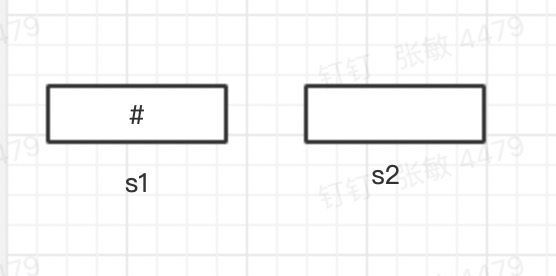1. 取字符
• 2 运算符 直接入 s2; / 操作符 入 s1;
• 8运算符 入 s2;
• ’ -'操作符 入 s1; 栈顶元素 / 优先级大于 - 所以取出/ 入s2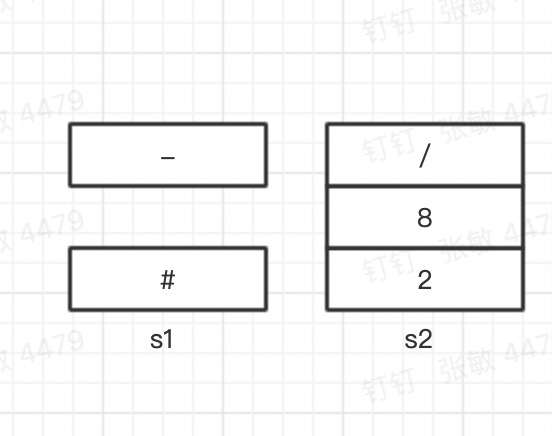- )操作符 入 s1;
-  )操作符 入 s1;
-  4 运算符 入 s2;
- '- 操作符 入 s1;
-  7运算符 入 s2;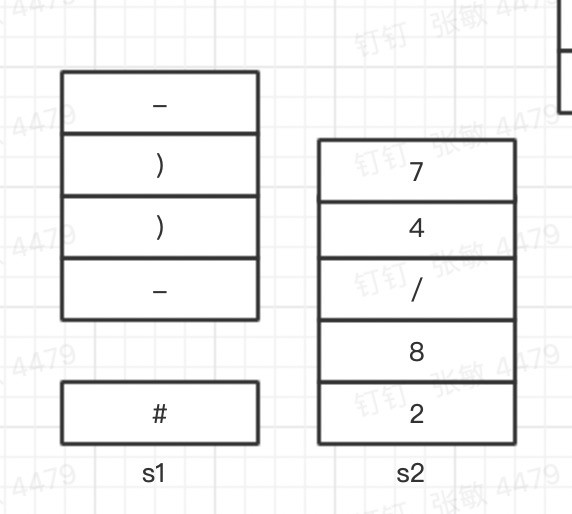• (操作符 将- 放入 s2 中, 移除 ( ) 两个;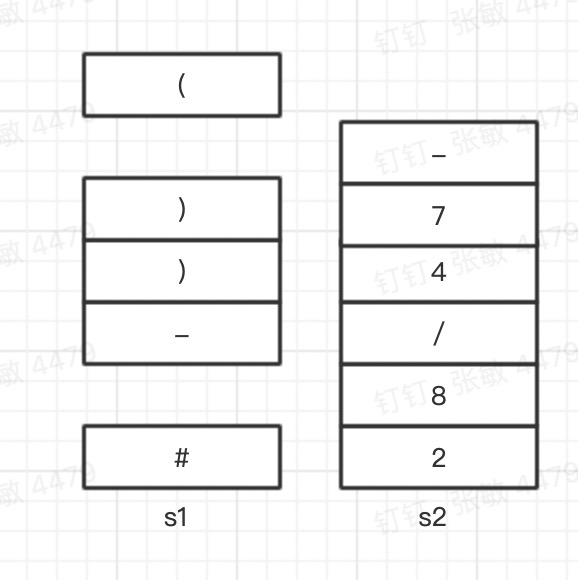• *操作符 入 s1;

• 3 运算符 入 s2;

• '+ 操作符 , 入s1, 但是 栈顶* 优先级大于 + 所以 * 出栈 入 s2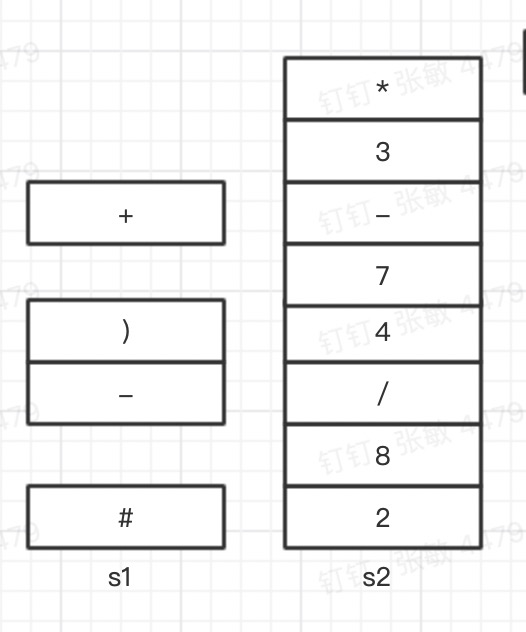• 6 运算符 入 s2;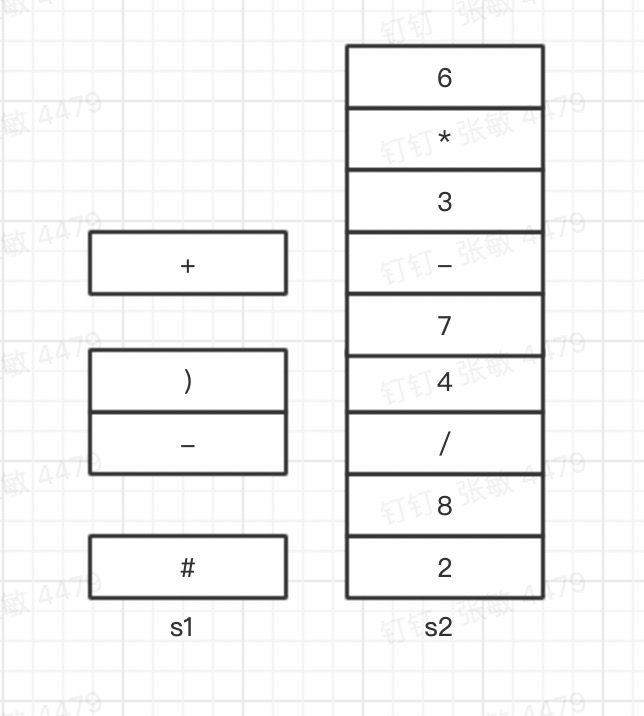• ( 取出 + 作废 ) ( ;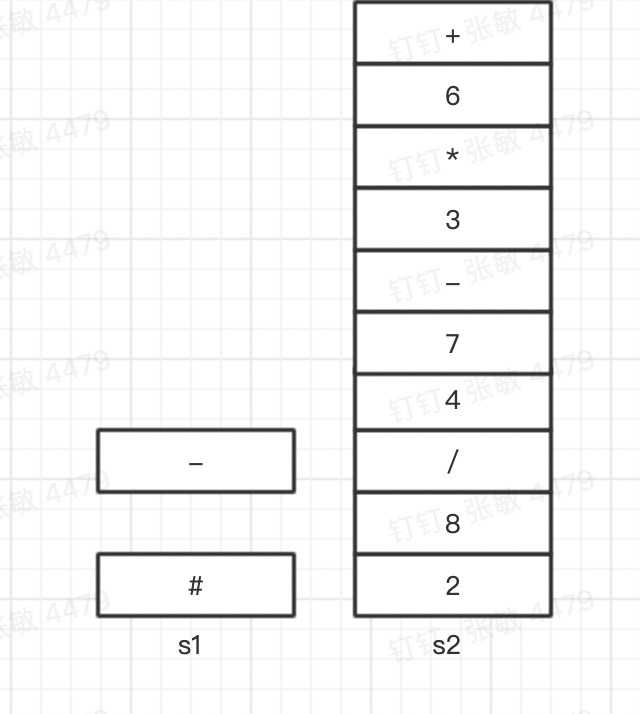• 结束 依次取出s1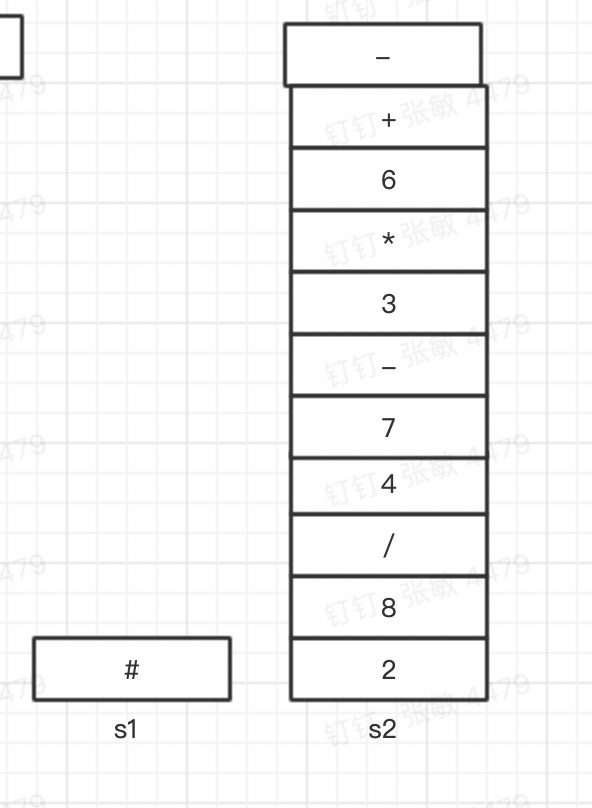逆缀 输出 - + 6 * 3-74/82

展开全文算法 数据结构
• 中缀表达式转前缀、后缀表达式

如果有实现四则混合运算这种需求，经常会遇到如何将中缀表达式转换为前缀或者后缀表达式的问题，在用代码实现转换时，一种常见的转换方式就是使用栈结构。

我特意整理了一个程序流程图，按照流程图写出程序就会简单很多，黑线部分就是程序的流程走向

中缀转前缀和中缀转后缀整体思路是一致的，只需要注意三个地方的区别即可

1.中缀转前缀是从右往左遍历表达式，中缀转后缀是从左往右遍历表达式；

2.运算符入栈时，对优先级的比较有所差异（中转前是≥ ；中转后是＞），具体看下面的流程图红色加粗部分

3.由于遍历顺序不同，对左右括号的处理也是相反的

区分前缀和后缀的遍历顺序，以及运算符入栈的优先级比较有所区别就行。

前提条件：

创建两个栈，一个用于存储运算符（"+"、"-"、"*"、"/"、"("、")"），命名为sign，一个用于存储操作数，命名为num。

转换完成后取出结果：

中缀转前缀：依次从栈顶遍历取出num栈的元素，即是前缀表达式

中缀转后缀：倒叙从栈底遍历取出num栈的元素，即是后缀表达式

中缀转前缀流程图如下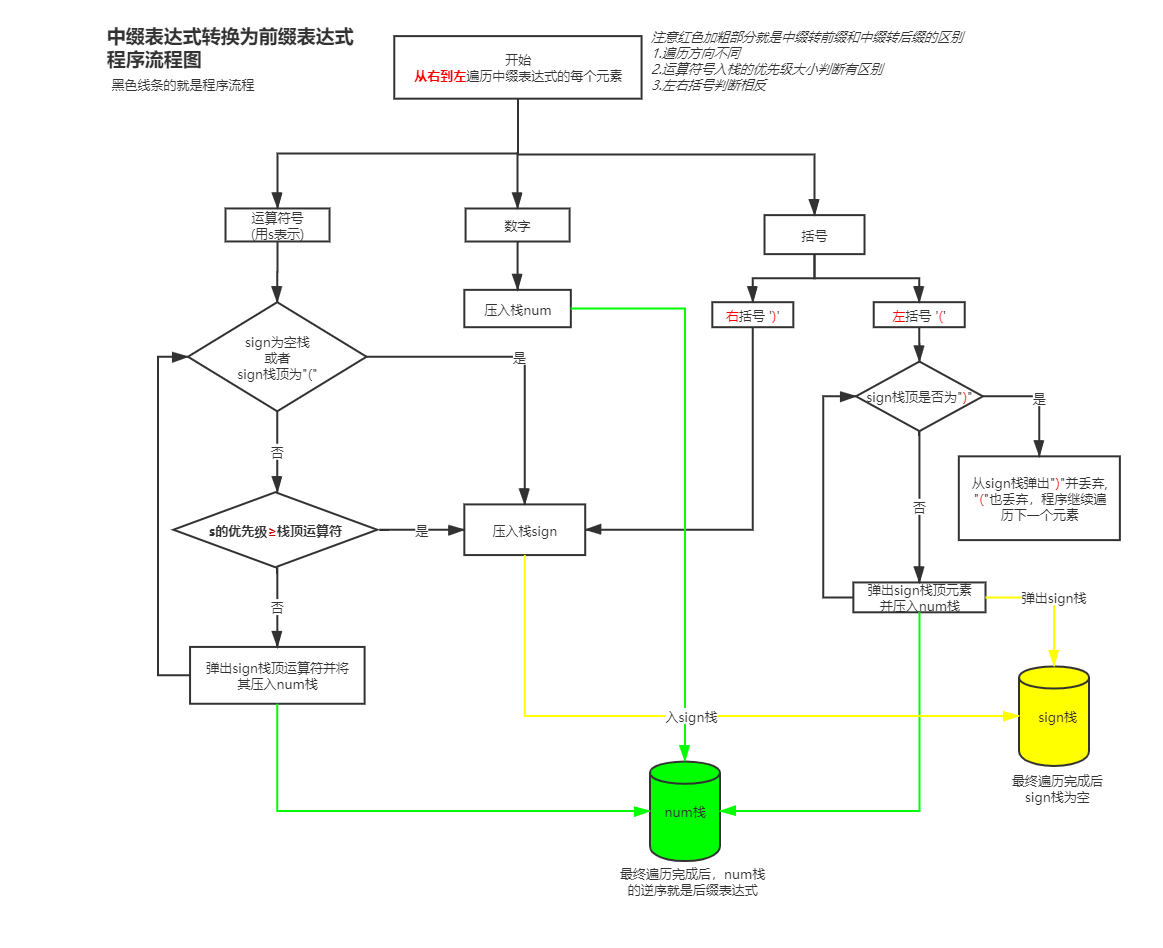走到最后，将如果sign中还有元素，就将其依次出栈然后入栈num，若没有元素就不管sign了

最后num栈依次出栈的就是所求前缀表达式

中缀转后缀流程图如下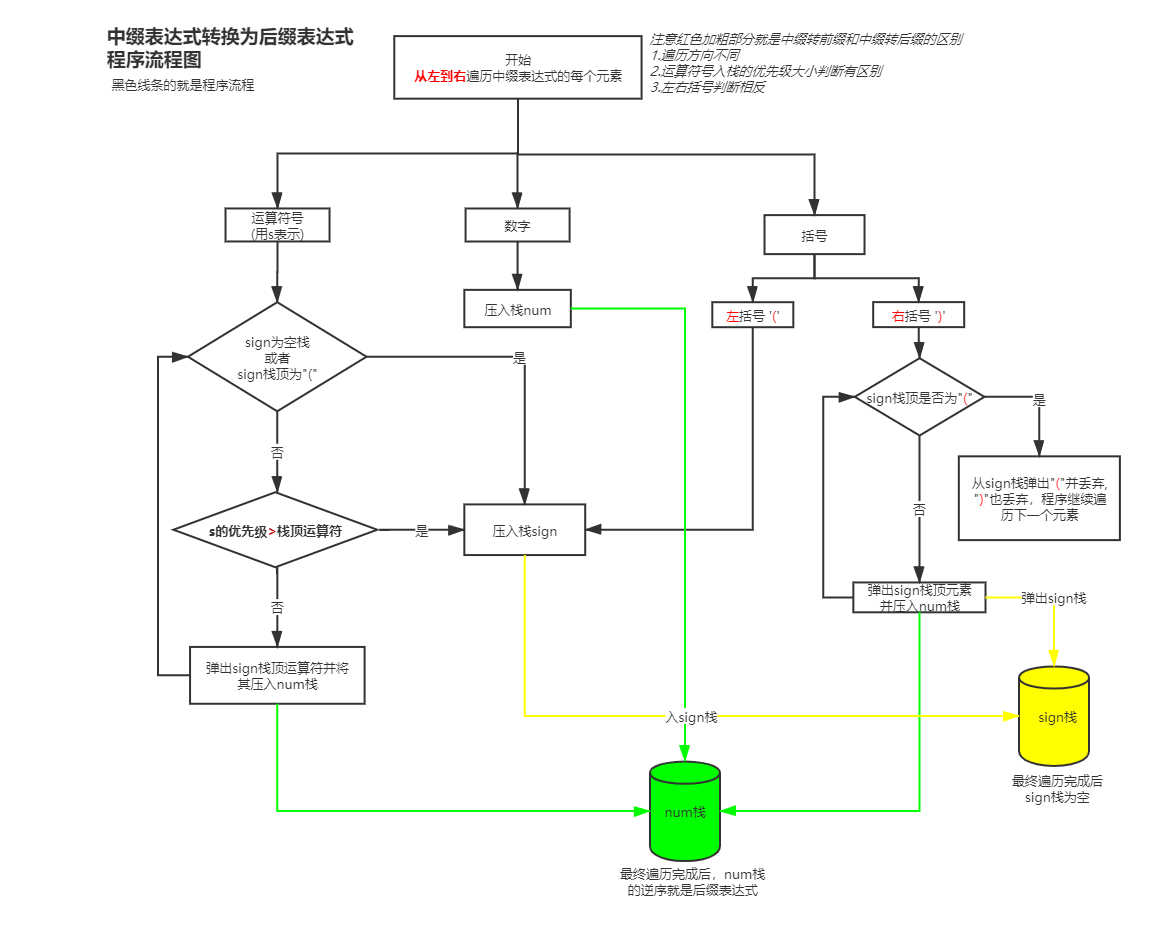走到最后，将如果sign中还有元素，就将其依次出栈然后入栈num，若没有元素就不管sign了

最后num栈逆序出栈的就是所求前缀表达式

具体代码实现如下，我只是通过流程图实现了代码，还没有对其做优化

计算器类Calculator

import java.util.*;

/**
*利用前缀、后缀表达式实现计算器计算个位数的四则混合运算
*/
public class Calculator {
public static final String PRE = "^[-+]?(([0-9]+)([.]([0-9]+))?|([.]([0-9]+))?)\$";//数字校验正则表达式
/**
* 传入表达式，计算并返回结果
* 返回的float类型只提供 -2^31 ~ 2^31-1范围内的运算
* @param expre 传入的表达式
* @param calcWay 计算方式：前缀后者后缀
* @return 计算结果
*/
public String calculation(String expre,String calcWay){
char[] exp ;
Stack<Float> calcStack = new Stack();
float re = 0;
if (calcWay.equals("Prefix") || calcWay == "Prefix") {
exp = convertToPrefix(expre);
float a,b;
//逆序遍历，遇到数字入栈，遇到符号取出栈顶两个元素计算并将结果再次入栈
for (int i = exp.length-1; i >=0; i--) {
if(exp[i] == '+' || exp[i] == '-' || exp[i] == '*' || exp[i] == '/'){
//取出栈顶两个元素并计算
a = calcStack.pop();
b = calcStack.pop();
switch (exp[i]){
//注意运算时，大数在前，小数在后，不然减法和乘法会计算错误
case '+':calcStack.push(a+b);break;
case '-':calcStack.push(a-b);break;
case '*':calcStack.push(a*b);break;
case '/':calcStack.push(a/b);break;
}
}else{
calcStack.push((float) exp[i] - '0');
}
}
re = calcStack.peek();//栈中最后一个元素即为计算结果
System.out.println("前缀表达式为"+String.valueOf(exp));
}
else if(calcWay.equals("Suffix") || calcWay == "Suffix"){
exp = convertToSuffix(expre);
System.out.println("后缀表达式为"+String.valueOf(exp));

float a,b;
for (int i = 0; i < exp.length; i++) {
if(exp[i] == '+' || exp[i] == '-' || exp[i] == '*' || exp[i] == '/'){
//取出栈顶两个元素并计算
a = calcStack.pop();
b = calcStack.pop();
switch (exp[i]){
//注意运算时，大数在前，小数在后，不然减法和乘法会计算错误
case '+':calcStack.push(b+a);break;
case '-':calcStack.push(b-a);break;
case '*':calcStack.push(b*a);break;
case '/':calcStack.push(b/a);break;
}
}else{
calcStack.push((float) exp[i] - '0');
}
}
re = calcStack.peek();//栈中最后一个元素即为计算结果
}
else {
throw new RuntimeException("计算方式只能为'Prefix'或者'Suffix'");
}

//计算完成后，若为正数，就去掉float后面的小数点和0
String result = (Math.round(re)-re==0) ? String.valueOf((long)re) : String.valueOf(re);
return result;
}

/**
* 转换为前缀表达式
* @param expre
* @return
*/
public char[] convertToPrefix(String expre){
char[] preFix = expre.toCharArray();
Stack<Character> sign = new Stack<>();
Stack<Character> num = new Stack<>();

for(int i = preFix.length-1;i>=0;i--){//从右到左遍历表达式
if(preFix[i] == ')'){
sign.push(preFix[i]);
}
else if(preFix[i] == '('){
if(sign.peek() == ')'){
sign.pop();//弹出'(' 丢弃
}else{
//遍历sign栈，并将其中的元素弹出压栈到num栈，直到找到左括号就弹出并结束
while (sign.size()>0){
if(sign.peek() == ')') {
sign.pop();//直到栈顶元素为 ')'结束并丢弃括号
break;
}
num.push(sign.pop());
}
}
}
else if(preFix[i] == '+' || preFix[i] == '-' || preFix[i] == '*' || preFix[i] == '/'){
if(sign.empty() || sign.peek() == ')'){//为空或顶部元素为')'就直接压栈
sign.push(preFix[i]);
}
else{
if(isHighOrEqualThan(preFix[i],sign.peek())){//当前的运算符比栈顶元素优先级高
sign.push(preFix[i]);
}
else{
while (true){
//遍历sign栈，将栈顶元素取出并压入num栈
// 直到遇到')'或者sign栈空 又或者找到一个元素优先级比当前元素高
if(sign.empty() || sign.peek() == ')' || isHighOrEqualThan(preFix[i],sign.peek())) {
sign.push(preFix[i]);
break;
}
num.push(sign.pop());
}
}
}
}
else if(String.valueOf(preFix[i]).matches(PRE)){  //正则验证是否为是数字，直接入栈
num.push(preFix[i]);
}
else{
throw new RuntimeException(preFix[i]+"不是数字和运算符，请检查输入的算式是否正确");
}
}
//遍历完成后，将sign剩余的所有符号依次出栈push到num栈中
while (sign.size()>0) num.push(sign.pop());

char[] result = new char[num.size()];
//逆序获取num栈并保存到结果数组
for(int forntIndex = 0;num.size()>0;forntIndex++){
result[forntIndex] = num.pop();
}
return result;
}

/**
* 转换为后缀表达式
* 转换流程图参考：https://blog.csdn.net/c_o_d_e_/article/details/108774118
* @param expre
* @return
*/
public char[] convertToSuffix(String expre){
char[] suffix = expre.toCharArray();
Stack<Character> sign = new Stack<>();
Stack<Character> num = new Stack<>();

for(int i = 0;i<suffix.length;i++){//从左到右遍历表达式
if(suffix[i] == '('){
sign.push(suffix[i]);
}
else if(suffix[i] == ')'){
if(sign.peek() == '('){
sign.pop();//弹出'(' 丢弃
}else{
//遍历sign栈，并将其中的元素弹出压栈到num栈，直到找到左括号就弹出并结束
while (sign.size()>0){
if(sign.peek() == '(') {
sign.pop();//直到栈顶元素为 '('结束并丢弃括号
break;
}
num.push(sign.pop());
}
}
}
else if(suffix[i] == '+' || suffix[i] == '-' || suffix[i] == '*' || suffix[i] == '/'){
if(sign.empty() || sign.peek() == '('){//为空或顶部元素为'('就直接压栈
sign.push(suffix[i]);
}
else{
if(isHighThan(suffix[i],sign.peek())){//当前的运算符比栈顶元素优先级高
sign.push(suffix[i]);
}
else{
while (true){
//遍历sign栈，将栈顶元素取出并压入num栈
// 直到遇到'('或者sign栈空 又或者找到一个元素优先级比当前元素高
if(sign.empty() || sign.peek() == '(' || isHighThan(suffix[i],sign.peek())) {
sign.push(suffix[i]);
break;
}
num.push(sign.pop());
}
}
}
}
else if(String.valueOf(suffix[i]).matches(PRE)){  //正则验证是否为是数字，直接入栈
num.push(suffix[i]);
}
else{
throw new RuntimeException(suffix[i]+"不是数字和运算符，请检查输入的算式是否正确");
}
}
//遍历完成后，将sign剩余的所有符号依次出栈push到num栈中
while (sign.size()>0) num.push(sign.pop());

char[] result = new char[num.size()];
//逆序获取num栈并保存到结果数组
for(int lastIndex = result.length-1;num.size()>0;lastIndex--){
result[lastIndex] = num.pop();
}
return result;
}

/**
* 比较两个运算符的优先级signOb是否高于compareOb
* @param signOb 要比较的符号
* @param compareOb 比较的对象
* @return true表示sign优先级高于compareOb
*/
public static boolean isHighThan(char signOb,char compareOb){
if((signOb == '+' || signOb == '-') && (compareOb == '*' || compareOb == '/'))//低于
return false;
else if((signOb == '+' || signOb == '-') && (compareOb == '+' || compareOb == '-')) //相同
return false;
else if((signOb == '*' || signOb == '/') && (compareOb == '*' || compareOb == '/')) //相同
return false;
else if((signOb == '*' || signOb == '/') && (compareOb == '+' || compareOb == '-')) //高于
return true;
else
throw  new RuntimeException("该符号不是加减乘除：sign:"+signOb+",compareOb:"+compareOb);
}

/**
* 比较两个运算符的优先级signOb是否高于或等于compareOb
* @param signOb 要比较的符号
* @param compareOb 比较的对象
* @return true表示sign优先级高于compareOb
*/
public static boolean isHighOrEqualThan(char signOb,char compareOb){
if((signOb == '+' || signOb == '-') && (compareOb == '*' || compareOb == '/'))//低于
return false;
else if((signOb == '+' || signOb == '-') && (compareOb == '+' || compareOb == '-')) //相同
return true;
else if((signOb == '*' || signOb == '/') && (compareOb == '*' || compareOb == '/')) //相同
return true;
else if((signOb == '*' || signOb == '/') && (compareOb == '+' || compareOb == '-')) //高于
return true;
else
throw  new RuntimeException("该符号不是加减乘除：sign:"+signOb+",compareOb:"+compareOb);
}
}


测试类Main

import java.util.*;
public class Main {
public static void main(String args[]){
System.out.println("请输出四则混合运算表达式（仅限于个位数）：");
Calculator calc = new Calculator();
Scanner scn = new Scanner(System.in);
while(scn.hasNext()){
String expres = scn.nextLine();
System.out.println("请输入计算方式，Prefix(前缀)或Suffix(后缀)：");
String fix = scn.nextLine();
System.out.println(calc.calculation(expres,fix));
}
}
}

测试结果如下图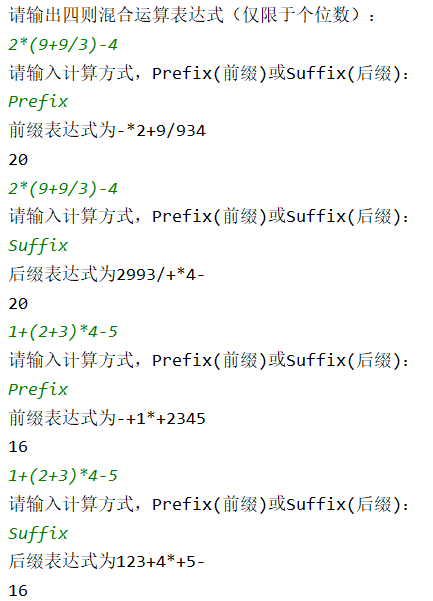展开全文数据结构 算法 程序设计
• 中缀表达式就是我们数学中常用的那种表达式，本文打算以中缀表达式：1 + (2 + 3) × 4 - 5 为例，分享一下前缀表达式和后缀表达式的如何形成与在计算机如何计算前后最表达式和后缀表达式 1、前缀表达式和后缀表达式...

目录

1、前缀表达式和后缀表达式的形成

1.1、中缀表达式转前缀表达式

1.2、中缀表达式转后缀表达式

1.3、注意点  （重点）

2、前缀表达式和后缀表达式在计算机中如何计算。

2.1、计算前缀表达式的值

2.2、计算后缀表达式的值

中缀表达式就是我们数学中常用的那种表达式，本文打算以中缀表达式：1 + (2 + 3) × 4 - 5  为例，分享一下前缀表达式和后缀表达式的如何形成与在计算机如何计算前后最表达式和后缀表达式

# 1、前缀表达式和后缀表达式的形成

前缀表达式和后缀表达式中的这个“缀”就是指符号的移动方向（通俗来说，就是符号统一朝哪边靠）

前缀表达式和后缀表达式形成都是对于整个中缀表达式按照运算顺序见运算符就加括号，保证每个运算符外面一定要有括号（原本有就没必要加了），然后每个符号挪到自己那层括号的外面（前缀是前面，后缀是后面），然后去掉括号。

中缀表达式：1 + (2 + 3) × 4 - 5

((1 +((2 + 3) × 4)）- 5）

前缀表达式：-  (+(1 ×(+(2 3)  4)）5），去掉括号得：  - +1×+2345

后缀表达式： ((1 ((2 3) + 4)×）+5)-，去掉括号得： 123+4×+5-

这样前缀表达式和后缀表达式就出来了。

那如何在机器层面将中缀表达式变成后缀表达式和前缀表达式

## 1.1、中缀表达式转前缀表达式

1）设置一个符号栈S，一个数字栈D

2）从中缀表达式右边开始遍历

3）遇到数字，压入数字栈D

遇到右括号，压入符号栈S

遇到运算符，如果符号栈栈顶为右括号，当前运算符直接入符号栈

如果符号栈栈顶运算符优先级小于等于当前运算符，当前运算符也直接入符号栈

如果符号栈栈顶运算符优先级大于当前运算符，那就栈顶元素出栈然后入栈数字栈，接着继续比较，直                                  到栈顶元素为右括号或者优先级小于等于当前运算符的运算符。

遇到左括号，符号栈开始出栈，出栈元素依次进入数字栈，直到遇见右括号为止（右括号不入数字栈，和左括号一起删掉）

图示过程如下（中缀表达式：1 + (2 + 3) × 4 - 5）：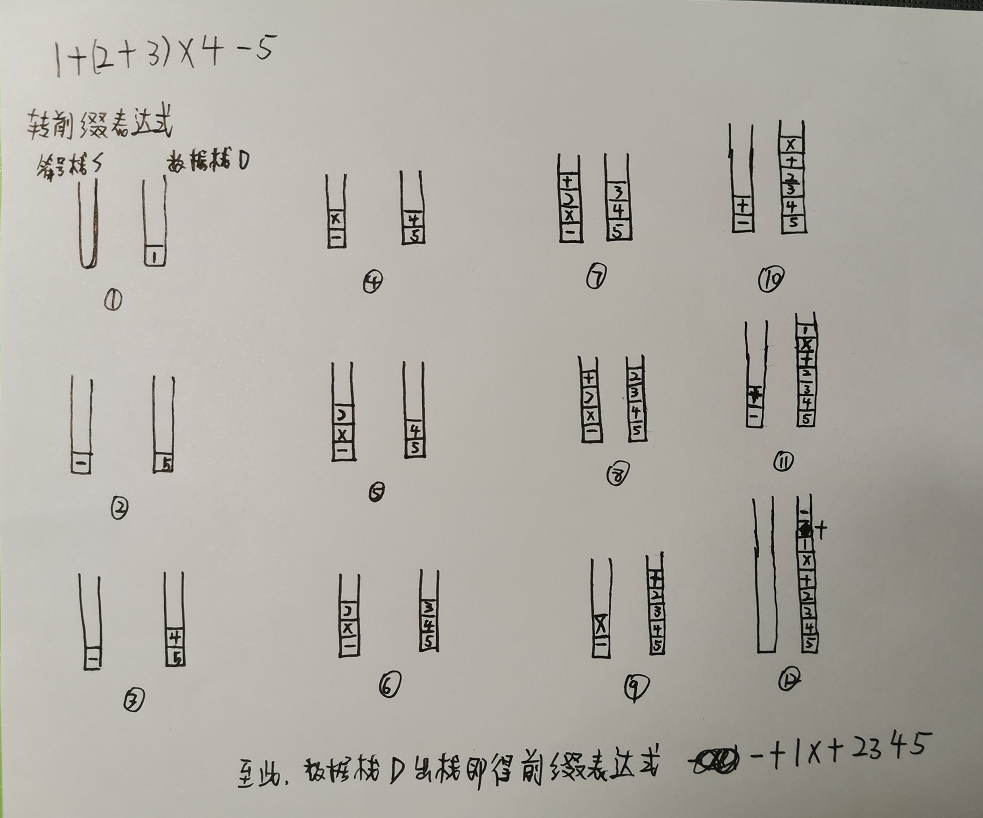## 1.2、中缀表达式转后缀表达式

1、设置一个符号栈S，一个队列Q

2、从中缀表达式左边开始遍历

3、遇到数字，入队列D

遇到左括号，压入符号栈S

遇到运算符，如果符号栈栈顶为左括号，当前运算符直接入符号栈

如果符号栈栈顶运算符优先级小于当前运算符，当前运算符也直接入栈

如果符号栈栈顶运算符优先级大于等于当前运算符，那就栈顶元素出栈然后入栈数字栈，接着继续比较，直                         到栈顶元素为右括号或者优先级小于当前运算符的运算符。

遇到右括号括号，符号栈开始出栈，出栈元素依次进入数字栈，直到遇见左括号为止（右括号不入队列，和左括号一起删掉）

图示过程如下（中缀表达式：1 + (2 + 3) × 4 - 5）：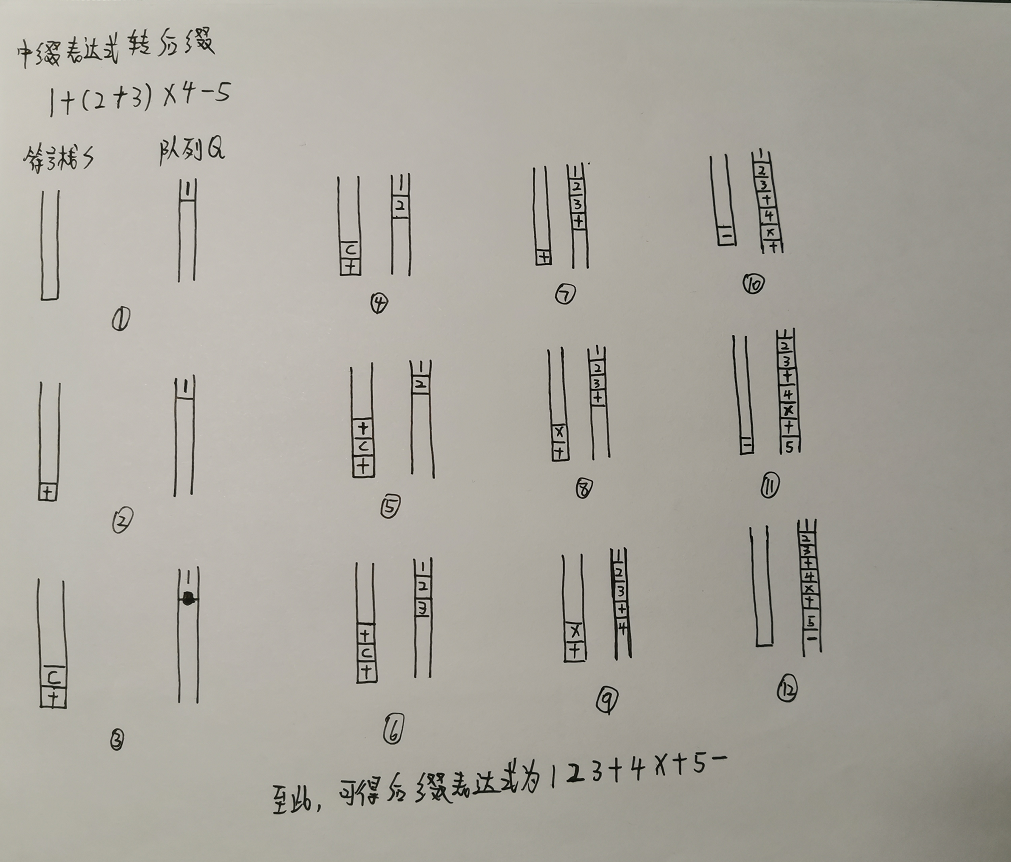## 1.3、注意点  （重点）

中缀表达式转前缀表达式的时候，在比较运算符的优先级的时候，同优先级的不出栈

中缀表达式转后缀表达式的时候，在比较运算符的优先级的时候，同优先级的出栈

是因为前缀表达式是从后往前去解析，同优先级下先入栈的应该后运算，所以不需要出栈（判断是大于即可），后面一起出栈是为了保证按照原本顺序运算。

而后缀表达式本身顺序就是按照从前往后解析，同优先级下先入栈的应该先出栈运算，所以需要出栈（是判断大于等于才行）。

# 2、前缀表达式和后缀表达式在计算机中如何计算。

## 2.1、计算前缀表达式的值

前缀表达式：  - +1×+2345。前缀表达式从后向前读取表达式，遇到数字就压入栈，遇到符号就弹出两个数字用当前运算符进行操作。

- +1×+2345

 剩余表达式 当前元素 栈 值 - +1×+234 5 5 NULL - +1×+23 4 45 NULL - +1×+2 3 345 NULL - +1×+ 2 2345 NULL - +1× + 45 2+3 = 5 - +1 × 5 5*4 = 20 - + 1 15 20 - + 5 20+1= 21 - 21-5 = 16

## 2.2、计算后缀表达式的值

后缀表达式： 123+4×+5-。后缀表达式从前向后读取表达式，遇到数字就压入栈，遇到符号就弹出两个数字用当前运算符进行操作。

你，总要埋头去做一些事情，不是吗

展开全文数据结构
• 文章目录前缀表达式（波兰表达式）前缀表达式分析与介绍思路分析中缀表达式中缀表达式分析与介绍后缀表达式（逆波兰表达式）后缀表达式分析与介绍思路分析逆波兰计算器代码实现逆波兰计算器中缀表达式转换为后缀...
• 刚刚做完中缀表达式转前缀表达式，想着试试看能不能把中缀表达式转化为前缀表达式，我自己笨想不来怎么做，然后就在网上找了很多教程，看看原理以及思路。 找了一个最笨的解决方法就是先逆序读中缀表达式，然后照着...指针 数据结构 程序设计 堆栈
• 中缀表达式转前缀表达式java实现 准备知识： 前缀、中缀、后缀表达式 http://note.youdao.com/noteshare?id=c27a0ee6222c18ec60cf5bb2154b0744&sub=4CEC6E1E196B4B228839B9220F9F673C 前缀、中缀、后缀表达式和...
• 3、从右至左扫描中缀表达式，如果是数字，就直接压入结果栈 若是运算符，则与运算符栈顶元素比较优先级：若该运算符优先级大于等于栈顶元素，则将该运算符入栈 否则栈内元素出栈并压入结果栈，再与其比较，直到该...
• /** 北理2013年第三题  2018-8-15 13:42 输入表达式，输出相应二叉树的前序遍历结果 ...(2) 从右至左扫描中缀表达式； (3) 遇到操作数时，将其压入S2； (4) 遇到运算符时，比较其与S1栈顶运算符的优先级：  ...stack
• 本文主要介绍中缀表达式转前缀表达式算法和代码实现数据结构 算法
• 这是一个循环♻️过程 4）辅助栈的指针前移一位（这是因为当前指在右括号）， 5）若扫描完表达式之后，辅助栈还有元素，全部出栈，入到结果栈 此时，将中间结果栈的元素全部出栈，得到的就是前缀表达式
• 2、利用栈实现中缀表达式前缀表达式的转换。 三、相关内容介绍 标准的表达式如"A+B"，在数学上学名叫中缀表达式（Infix Notation），原因是运算符号在两个运算对象的中间。相对应的还有前缀表达式（Prefix ...
• 接上篇数据结构——栈（中缀表达式转后缀表达式） 这里再做一个前缀表达式的总结： 初始化两个栈：运算符栈S1和储存中间结果的栈S2； 从右至左扫描中缀表达式； 遇到操作数时，将其压入S2； 遇到运算符时，比较其与...java 数据结构
• 中缀表达式转前缀、后缀表达式，并计算其值2.1 中缀表达式转后缀表达式2.1.1 思路2.1.2 代码实现：2.2 中缀表达式转前缀表达式2.2.1 思路2.2.2 代码实现： 1.几种表达式的了解 1.1中缀表达式 1.1.1 定义 所谓的中缀...数据结构 java
• 作完了中缀前缀，作一个归纳吧。 https://www.cnblogs.com/unixfy/p/3344550.html # coding = utf-8 class Stack: def __init__(self): self.items = [] # 是否为空 def is_empty(self): ...
• 目的将中缀表达式（即标准的表达式）转换为前缀表达式例如：1+2*3+(4*5+6)7 转换成 ++1*23+*4567转换原则：与中缀后缀不同，前者是顺序从左到右读取每一个字符，后者是从右到左顺序读取每一个字符，然后进行反转...
• 中缀表达式转前/后缀表达式-利用栈(C语言）中缀表达式转前缀表达式规则中缀表达式转后缀表达式规则 中缀表达式转前缀表达式规则 使用两个栈，运算符栈，数字(操作数)栈. 从右到左遍历表达式. 遇到操作数时，直接放...数据结构 算法
• 逆波兰式（字符栈） 自 https://www.cnblogs.com/unixfy/p/3344550.html  栈高栈出 栈小外进 同号栈高 左括必进遇右出 #include #include #include int main() { int i; char str; char seqstack; ...
• 中缀表达式转后缀表达式 方法一：括号法 ①按照运算符的优先级，对所有的运算单位加括号。 于是变成：(((a+b)-(a*(((c+d)/e)-f)))+g) ②从最里面的括号开始，依次把运算符号移动到对应的括号的后面。 于是变成...c语言
• 中缀表达式转前缀表达式求值 首先将中缀表达式转换成前缀表达式 前缀表达式中，操作符在前 例如：1+2*(5-3)+4 后缀表达式：++1*2-534 一、转换思路 转换思路为将输入的中缀表达式字符串从右往左扫描(字符前后加#号)...数据结构 算法
• 该程序实现了运算表达式转换为中缀表达式中缀表达式转换为后缀表达式及后缀表达式求值。该程序已实现加减乘除括号运算符及求余、幂指数的求解
• 一、中缀表达式转后缀表达式这里的运算思路跟我代码一样，所以我就直接借鉴的别人的逆波兰表达式又称作后缀表达式，在四则混合运算的程序设计中用到。例如：1+2写成后缀表达式就是12+4+5*(3-2)的后缀表达式就是4532-...
• * 中缀表达式转前缀表达式求解 * ( 3 + 4 ) × 5 - 6 * 输入一个等式操作数与操作符用空格隔开 * */ public class ClassA { //定义所有的操作符及其优先级 static String opp = ")+-*/(";
• 中缀转后缀表达式考虑表达式 A + B * C。A B C * +是等价的后缀表达式。 我们已经注意到，操作数 A，B 和 C 保持在它们的相对位置。只有操作符改变位置。再看中缀表达式中的运算符。从左到右出现的第一个运算符为 +...算法 后缀表达式 python
• 本文主要介绍中缀表达式转前缀表达式计算机求值算法和代码实现
• 中缀表达式： a+b*c-(d+e) 第一步：按照运算符的优先级对所有的运算单位加括号 式子变成拉：((a+(b*c))-(d+e)) 第二步：转换前缀与后缀表达式 前缀：把运算符号移动到对应的括号前面 则变成拉：-( +(a *...后缀表达式
• 1、从左往右扫描中缀表达式，如果是数字，写入结果表达式，如果是操作数，需要进一步判断 2、（1）如果是左括号’(’，直接入栈 （2）如果是运算符，（‘+’、‘-’、‘*’、‘/’），先判断栈顶的操作数的优先级，...数据结构...